# garage.torch.modules¶

PyTorch Modules.

class CategoricalCNNModule(input_var, output_dim, kernel_sizes, hidden_channels, strides=1, hidden_sizes=(32, 32), hidden_nonlinearity=torch.tanh, hidden_w_init=nn.init.xavier_uniform_, hidden_b_init=nn.init.zeros_, paddings=0, padding_mode='zeros', max_pool=False, pool_shape=None, pool_stride=1, output_nonlinearity=None, output_w_init=nn.init.xavier_uniform_, output_b_init=nn.init.zeros_, layer_normalization=False, is_image=True)

Bases: torch.nn.Module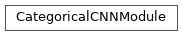Categorical CNN Model.

A model represented by a Categorical distribution which is parameterized by a convolutional neural network (CNN) followed by a fully-connected layer.

Parameters
• input_var (torch.tensor) – Input tensor of the model.

• output_dim (int) – Output dimension of the model.

• kernel_sizes (tuple[int]) – Dimension of the conv filters. For example, (3, 5) means there are two convolutional layers. The filter for first layer is of dimension (3 x 3) and the second one is of dimension (5 x 5).

• strides (tuple[int]) – The stride of the sliding window. For example, (1, 2) means there are two convolutional layers. The stride of the filter for first layer is 1 and that of the second layer is 2.

• hidden_channels (tuple[int]) – Number of output channels for CNN. For example, (3, 32) means there are two convolutional layers. The filter for the first conv layer outputs 3 channels and the second one outputs 32 channels.

• hidden_sizes (list[int]) – Output dimension of dense layer(s) for the MLP for mean. For example, (32, 32) means the MLP consists of two hidden layers, each with 32 hidden units.

• hidden_nonlinearity (callable) – Activation function for intermediate dense layer(s). It should return a torch.Tensor. Set it to None to maintain a linear activation.

• hidden_w_init (callable) – Initializer function for the weight of intermediate dense layer(s). The function should return a torch.Tensor.

• hidden_b_init (callable) – Initializer function for the bias of intermediate dense layer(s). The function should return a torch.Tensor.

• padding_mode (str) – The type of padding algorithm to use, either ‘SAME’ or ‘VALID’.

• max_pool (bool) – Bool for using max-pooling or not.

• pool_shape (tuple[int]) – Dimension of the pooling layer(s). For example, (2, 2) means that all the pooling layers are of the same shape (2, 2).

• pool_stride (tuple[int]) – The strides of the pooling layer(s). For example, (2, 2) means that all the pooling layers have strides (2, 2).

• output_nonlinearity (callable) – Activation function for output dense layer. It should return a torch.Tensor. Set it to None to maintain a linear activation.

• output_w_init (callable) – Initializer function for the weight of output dense layer(s). The function should return a torch.Tensor.

• output_b_init (callable) – Initializer function for the bias of output dense layer(s). The function should return a torch.Tensor.

• layer_normalization (bool) – Bool for using layer normalization or not.

• is_image (bool) – Whether observations are images or not.

forward(self, *inputs)

Forward method.

Parameters

*inputs – Input to the module.

Returns

Policy distribution.

Return type

torch.distributions.Categorical

class CNNModule(input_var, hidden_channels, kernel_sizes, strides, hidden_nonlinearity=nn.ReLU, hidden_w_init=nn.init.xavier_uniform_, hidden_b_init=nn.init.zeros_, paddings=0, padding_mode='zeros', max_pool=False, pool_shape=None, pool_stride=1, layer_normalization=False, n_layers=None, is_image=True)

Bases: torch.nn.Module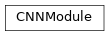Convolutional neural network (CNN) model in pytorch.

Parameters
• input_var (pytorch.tensor) – Input tensor of the model. Based on ‘NCHW’ data format: [batch_size, channel, height, width].

• kernel_sizes (tuple[int]) – Dimension of the conv filters. For example, (3, 5) means there are two convolutional layers. The filter for first layer is of dimension (3 x 3) and the second one is of dimension (5 x 5).

• strides (tuple[int]) – The stride of the sliding window. For example, (1, 2) means there are two convolutional layers. The stride of the filter for first layer is 1 and that of the second layer is 2.

• hidden_channels (tuple[int]) – Number of output channels for CNN. For example, (3, 32) means there are two convolutional layers. The filter for the first conv layer outputs 3 channels and the second one outputs 32 channels.

• hidden_nonlinearity (callable or torch.nn.Module) – Activation function for intermediate dense layer(s). It should return a torch.Tensor. Set it to None to maintain a linear activation.

• hidden_w_init (callable) – Initializer function for the weight of intermediate dense layer(s). The function should return a torch.Tensor.

• hidden_b_init (callable) – Initializer function for the bias of intermediate dense layer(s). The function should return a torch.Tensor.

• paddings (tuple[int]) – Amount of zero-padding added to both sides of the input of a conv layer.

• padding_mode (str) – The type of padding algorithm to use, i.e. ‘constant’, ‘reflect’, ‘replicate’ or ‘circular’ and by default is ‘zeros’.

• max_pool (bool) – Bool for using max-pooling or not.

• pool_shape (tuple[int]) – Dimension of the pooling layer(s). For example, (2, 2) means that all pooling layers are of the same shape (2, 2).

• pool_stride (tuple[int]) – The strides of the pooling layer(s). For example, (2, 2) means that all the pooling layers have strides (2, 2).

• layer_normalization (bool) – Bool for using layer normalization or not.

• n_layers (int) – number of convolutional layer.

• is_image (bool) – Whether observations are images or not.

forward(self, input_val)

Forward method.

Parameters

input_val (torch.Tensor) – Input values with (N, C, H, W) shape.

Returns

Output values

Return type

List[torch.Tensor]

class GaussianMLPIndependentStdModule(input_dim, output_dim, hidden_sizes=(32, 32), hidden_nonlinearity=torch.tanh, hidden_w_init=nn.init.xavier_uniform_, hidden_b_init=nn.init.zeros_, output_nonlinearity=None, output_w_init=nn.init.xavier_uniform_, output_b_init=nn.init.zeros_, learn_std=True, init_std=1.0, min_std=1e-06, max_std=None, std_hidden_sizes=(32, 32), std_hidden_nonlinearity=torch.tanh, std_hidden_w_init=nn.init.xavier_uniform_, std_hidden_b_init=nn.init.zeros_, std_output_nonlinearity=None, std_output_w_init=nn.init.xavier_uniform_, std_parameterization='exp', layer_normalization=False, normal_distribution_cls=Normal)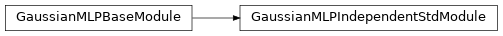GaussianMLPModule which has two different mean and std network.

Parameters
• input_dim (int) – Input dimension of the model.

• output_dim (int) – Output dimension of the model.

• hidden_sizes (list[int]) – Output dimension of dense layer(s) for the MLP for mean. For example, (32, 32) means the MLP consists of two hidden layers, each with 32 hidden units.

• hidden_nonlinearity (callable) – Activation function for intermediate dense layer(s). It should return a torch.Tensor. Set it to None to maintain a linear activation.

• hidden_w_init (callable) – Initializer function for the weight of intermediate dense layer(s). The function should return a torch.Tensor.

• hidden_b_init (callable) – Initializer function for the bias of intermediate dense layer(s). The function should return a torch.Tensor.

• output_nonlinearity (callable) – Activation function for output dense layer. It should return a torch.Tensor. Set it to None to maintain a linear activation.

• output_w_init (callable) – Initializer function for the weight of output dense layer(s). The function should return a torch.Tensor.

• output_b_init (callable) – Initializer function for the bias of output dense layer(s). The function should return a torch.Tensor.

• learn_std (bool) – Is std trainable.

• init_std (float) – Initial value for std. (plain value - not log or exponentiated).

• min_std (float) – If not None, the std is at least the value of min_std, to avoid numerical issues (plain value - not log or exponentiated).

• max_std (float) – If not None, the std is at most the value of max_std, to avoid numerical issues (plain value - not log or exponentiated).

• std_hidden_sizes (list[int]) – Output dimension of dense layer(s) for the MLP for std. For example, (32, 32) means the MLP consists of two hidden layers, each with 32 hidden units.

• std_hidden_nonlinearity (callable) – Nonlinearity for each hidden layer in the std network.

• std_hidden_w_init (callable) – Initializer function for the weight of hidden layer (s).

• std_hidden_b_init (callable) – Initializer function for the bias of intermediate dense layer(s).

• std_output_nonlinearity (callable) – Activation function for output dense layer in the std network. It should return a torch.Tensor. Set it to None to maintain a linear activation.

• std_output_w_init (callable) – Initializer function for the weight of output dense layer(s) in the std network.

• std_parameterization (str) –

How the std should be parametrized. There are two options: - exp: the logarithm of the std will be stored, and applied a

exponential transformation

• softplus: the std will be computed as log(1+exp(x))

• layer_normalization (bool) – Bool for using layer normalization or not.

• normal_distribution_cls (torch.distribution) – normal distribution class to be constructed and returned by a call to forward. By default, is torch.distributions.Normal.

to(self, *args, **kwargs)

Move the module to the specified device.

Parameters
• *args – args to pytorch to function.

• **kwargs – keyword args to pytorch to function.

forward(self, *inputs)

Forward method.

Parameters

*inputs – Input to the module.

Returns

Independent

distribution.

Return type

torch.distributions.independent.Independent

class GaussianMLPTwoHeadedModule(input_dim, output_dim, hidden_sizes=(32, 32), hidden_nonlinearity=torch.tanh, hidden_w_init=nn.init.xavier_uniform_, hidden_b_init=nn.init.zeros_, output_nonlinearity=None, output_w_init=nn.init.xavier_uniform_, output_b_init=nn.init.zeros_, learn_std=True, init_std=1.0, min_std=1e-06, max_std=None, std_parameterization='exp', layer_normalization=False, normal_distribution_cls=Normal)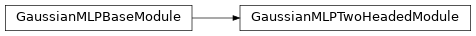GaussianMLPModule which has only one mean network.

Parameters
• input_dim (int) – Input dimension of the model.

• output_dim (int) – Output dimension of the model.

• hidden_sizes (list[int]) – Output dimension of dense layer(s) for the MLP for mean. For example, (32, 32) means the MLP consists of two hidden layers, each with 32 hidden units.

• hidden_nonlinearity (callable) – Activation function for intermediate dense layer(s). It should return a torch.Tensor. Set it to None to maintain a linear activation.

• hidden_w_init (callable) – Initializer function for the weight of intermediate dense layer(s). The function should return a torch.Tensor.

• hidden_b_init (callable) – Initializer function for the bias of intermediate dense layer(s). The function should return a torch.Tensor.

• output_nonlinearity (callable) – Activation function for output dense layer. It should return a torch.Tensor. Set it to None to maintain a linear activation.

• output_w_init (callable) – Initializer function for the weight of output dense layer(s). The function should return a torch.Tensor.

• output_b_init (callable) – Initializer function for the bias of output dense layer(s). The function should return a torch.Tensor.

• learn_std (bool) – Is std trainable.

• init_std (float) – Initial value for std. (plain value - not log or exponentiated).

• min_std (float) – If not None, the std is at least the value of min_std, to avoid numerical issues (plain value - not log or exponentiated).

• max_std (float) – If not None, the std is at most the value of max_std, to avoid numerical issues (plain value - not log or exponentiated).

• std_parameterization (str) –

How the std should be parametrized. There are two options: - exp: the logarithm of the std will be stored, and applied a

exponential transformation

• softplus: the std will be computed as log(1+exp(x))

• layer_normalization (bool) – Bool for using layer normalization or not.

• normal_distribution_cls (torch.distribution) – normal distribution class to be constructed and returned by a call to forward. By default, is torch.distributions.Normal.

to(self, *args, **kwargs)

Move the module to the specified device.

Parameters
• *args – args to pytorch to function.

• **kwargs – keyword args to pytorch to function.

forward(self, *inputs)

Forward method.

Parameters

*inputs – Input to the module.

Returns

Independent

distribution.

Return type

torch.distributions.independent.Independent

class GaussianMLPModule(input_dim, output_dim, hidden_sizes=(32, 32), hidden_nonlinearity=torch.tanh, hidden_w_init=nn.init.xavier_uniform_, hidden_b_init=nn.init.zeros_, output_nonlinearity=None, output_w_init=nn.init.xavier_uniform_, output_b_init=nn.init.zeros_, learn_std=True, init_std=1.0, min_std=1e-06, max_std=None, std_parameterization='exp', layer_normalization=False, normal_distribution_cls=Normal)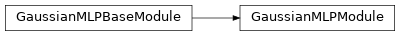GaussianMLPModule that mean and std share the same network.

Parameters
• input_dim (int) – Input dimension of the model.

• output_dim (int) – Output dimension of the model.

• hidden_sizes (list[int]) – Output dimension of dense layer(s) for the MLP for mean. For example, (32, 32) means the MLP consists of two hidden layers, each with 32 hidden units.

• hidden_nonlinearity (callable) – Activation function for intermediate dense layer(s). It should return a torch.Tensor. Set it to None to maintain a linear activation.

• hidden_w_init (callable) – Initializer function for the weight of intermediate dense layer(s). The function should return a torch.Tensor.

• hidden_b_init (callable) – Initializer function for the bias of intermediate dense layer(s). The function should return a torch.Tensor.

• output_nonlinearity (callable) – Activation function for output dense layer. It should return a torch.Tensor. Set it to None to maintain a linear activation.

• output_w_init (callable) – Initializer function for the weight of output dense layer(s). The function should return a torch.Tensor.

• output_b_init (callable) – Initializer function for the bias of output dense layer(s). The function should return a torch.Tensor.

• learn_std (bool) – Is std trainable.

• init_std (float) – Initial value for std. (plain value - not log or exponentiated).

• min_std (float) – If not None, the std is at least the value of min_std, to avoid numerical issues (plain value - not log or exponentiated).

• max_std (float) – If not None, the std is at most the value of max_std, to avoid numerical issues (plain value - not log or exponentiated).

• std_parameterization (str) –

How the std should be parametrized. There are two options: - exp: the logarithm of the std will be stored, and applied a

exponential transformation

• softplus: the std will be computed as log(1+exp(x))

• layer_normalization (bool) – Bool for using layer normalization or not.

• normal_distribution_cls (torch.distribution) – normal distribution class to be constructed and returned by a call to forward. By default, is torch.distributions.Normal.

to(self, *args, **kwargs)

Move the module to the specified device.

Parameters
• *args – args to pytorch to function.

• **kwargs – keyword args to pytorch to function.

forward(self, *inputs)

Forward method.

Parameters

*inputs – Input to the module.

Returns

Independent

distribution.

Return type

torch.distributions.independent.Independent

class MLPModule(input_dim, output_dim, hidden_sizes, hidden_nonlinearity=F.relu, hidden_w_init=nn.init.xavier_normal_, hidden_b_init=nn.init.zeros_, output_nonlinearity=None, output_w_init=nn.init.xavier_normal_, output_b_init=nn.init.zeros_, layer_normalization=False)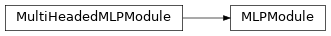MLP Model.

A Pytorch module composed only of a multi-layer perceptron (MLP), which maps real-valued inputs to real-valued outputs.

Parameters
• input_dim (int) – Dimension of the network input.

• output_dim (int) – Dimension of the network output.

• hidden_sizes (list[int]) – Output dimension of dense layer(s). For example, (32, 32) means this MLP consists of two hidden layers, each with 32 hidden units.

• hidden_nonlinearity (callable or torch.nn.Module) – Activation function for intermediate dense layer(s). It should return a torch.Tensor. Set it to None to maintain a linear activation.

• hidden_w_init (callable) – Initializer function for the weight of intermediate dense layer(s). The function should return a torch.Tensor.

• hidden_b_init (callable) – Initializer function for the bias of intermediate dense layer(s). The function should return a torch.Tensor.

• output_nonlinearity (callable or torch.nn.Module) – Activation function for output dense layer. It should return a torch.Tensor. Set it to None to maintain a linear activation.

• output_w_init (callable) – Initializer function for the weight of output dense layer(s). The function should return a torch.Tensor.

• output_b_init (callable) – Initializer function for the bias of output dense layer(s). The function should return a torch.Tensor.

• layer_normalization (bool) – Bool for using layer normalization or not.

forward(self, input_value)

Forward method.

Parameters

input_value (torch.Tensor) – Input values with (N, *, input_dim) shape.

Returns

Output value

Return type

torch.Tensor

property output_dim(self)

Return output dimension of network.

Returns

Output dimension of network.

Return type

int

class MultiHeadedMLPModule(n_heads, input_dim, output_dims, hidden_sizes, hidden_nonlinearity=torch.relu, hidden_w_init=nn.init.xavier_normal_, hidden_b_init=nn.init.zeros_, output_nonlinearities=None, output_w_inits=nn.init.xavier_normal_, output_b_inits=nn.init.zeros_, layer_normalization=False)

Bases: torch.nn.Module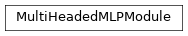A PyTorch module composed only of a multi-layer perceptron (MLP) with multiple parallel output layers which maps real-valued inputs to real-valued outputs. The length of outputs is n_heads and shape of each output element is depend on each output dimension

Parameters
• n_heads (int) – Number of different output layers

• input_dim (int) – Dimension of the network input.

• output_dims (int or list or tuple) – Dimension of the network output.

• hidden_sizes (list[int]) – Output dimension of dense layer(s). For example, (32, 32) means this MLP consists of two hidden layers, each with 32 hidden units.

• hidden_nonlinearity (callable or torch.nn.Module or list or tuple) – Activation function for intermediate dense layer(s). It should return a torch.Tensor. Set it to None to maintain a linear activation.

• hidden_w_init (callable) – Initializer function for the weight of intermediate dense layer(s). The function should return a torch.Tensor.

• hidden_b_init (callable) – Initializer function for the bias of intermediate dense layer(s). The function should return a torch.Tensor.

• output_nonlinearities (callable or torch.nn.Module or list or tuple) – Activation function for output dense layer. It should return a torch.Tensor. Set it to None to maintain a linear activation. Size of the parameter should be 1 or equal to n_head

• output_w_inits (callable or list or tuple) – Initializer function for the weight of output dense layer(s). The function should return a torch.Tensor. Size of the parameter should be 1 or equal to n_head

• output_b_inits (callable or list or tuple) – Initializer function for the bias of output dense layer(s). The function should return a torch.Tensor. Size of the parameter should be 1 or equal to n_head

• layer_normalization (bool) – Bool for using layer normalization or not.

forward(self, input_val)

Forward method.

Parameters

input_val (torch.Tensor) – Input values with (N, *, input_dim) shape.

Returns

Output values

Return type

List[torch.Tensor]

class DiscreteCNNModule(input_shape, output_dim, kernel_sizes, hidden_channels, strides, hidden_sizes=(32, 32), cnn_hidden_nonlinearity=nn.ReLU, mlp_hidden_nonlinearity=nn.ReLU, hidden_w_init=nn.init.xavier_uniform_, hidden_b_init=nn.init.zeros_, paddings=0, padding_mode='zeros', max_pool=False, pool_shape=None, pool_stride=1, output_nonlinearity=None, output_w_init=nn.init.xavier_uniform_, output_b_init=nn.init.zeros_, layer_normalization=False, is_image=True)

Bases: torch.nn.Module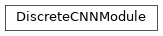Discrete CNN Module.

A CNN followed by one or more fully connected layers with a set number of discrete outputs.

Parameters
• input_shape (tuple[int]) – Shape of the input. Based on ‘NCHW’ data format: [batch_size, channel, height, width].

• output_dim (int) – Output dimension of the fully-connected layer.

• kernel_sizes (tuple[int]) – Dimension of the conv filters. For example, (3, 5) means there are two convolutional layers. The filter for first layer is of dimension (3 x 3) and the second one is of dimension (5 x 5).

• strides (tuple[int]) – The stride of the sliding window. For example, (1, 2) means there are two convolutional layers. The stride of the filter for first layer is 1 and that of the second layer is 2.

• hidden_channels (tuple[int]) – Number of output channels for CNN. For example, (3, 32) means there are two convolutional layers. The filter for the first conv layer outputs 3 channels and the second one outputs 32 channels.

• hidden_sizes (list[int]) – Output dimension of dense layer(s) for the MLP for mean. For example, (32, 32) means the MLP consists of two hidden layers, each with 32 hidden units.

• mlp_hidden_nonlinearity (callable) – Activation function for intermediate dense layer(s) in the MLP. It should return a torch.Tensor. Set it to None to maintain a linear activation.

• cnn_hidden_nonlinearity (callable) – Activation function for intermediate CNN layer(s). It should return a torch.Tensor. Set it to None to maintain a linear activation.

• hidden_w_init (callable) – Initializer function for the weight of intermediate dense layer(s). The function should return a torch.Tensor.

• hidden_b_init (callable) – Initializer function for the bias of intermediate dense layer(s). The function should return a torch.Tensor.

• padding_mode (str) – The type of padding algorithm to use, either ‘SAME’ or ‘VALID’.

• max_pool (bool) – Bool for using max-pooling or not.

• pool_shape (tuple[int]) – Dimension of the pooling layer(s). For example, (2, 2) means that all the pooling layers are of the same shape (2, 2).

• pool_stride (tuple[int]) – The strides of the pooling layer(s). For example, (2, 2) means that all the pooling layers have strides (2, 2).

• output_nonlinearity (callable) – Activation function for output dense layer. It should return a torch.Tensor. Set it to None to maintain a linear activation.

• output_w_init (callable) – Initializer function for the weight of output dense layer(s). The function should return a torch.Tensor.

• output_b_init (callable) – Initializer function for the bias of output dense layer(s). The function should return a torch.Tensor.

• layer_normalization (bool) – Bool for using layer normalization or not.

• is_image (bool) – If true, the inputs are normalized by dividing by 255.

forward(self, inputs)

Forward method.

Parameters

inputs (torch.Tensor) – Inputs to the model of shape (input_shape*).

Returns

Output tensor of shape $$(N, output_dim)$$.

Return type

torch.Tensor